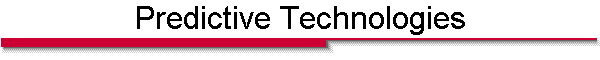Key Predictive Dynamix Modeling Algorithms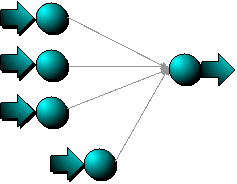Regression Models Regression models have been the mainstay of predictive modeling for many decades.  Linear regression models characterize the relationship between inputs and outputs using a linear equation (y = SXiWi + c) with one coefficient per input.  Regression coefficients are determined via a least squares algorithm to minimize error across the training dataset.Because there is a single weight per input linear regression models are easily interpreted but cannot represent non-linear relationships and interactions between inputs.  In order to extend the capabilities of regression models,  non-linear transformations are often applied to the model inputs or outputs (i.e., logistic regression, polynomial regression, etc.).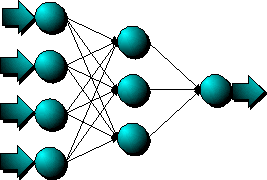Neural Networks Modern neural network technology are powerful computational structures for solving difficult problems involving forecasting and pattern recognition.   Multi-layer perceptron neural networks have an architecture that allows multiple coefficients per input variable.  The processing at each node is functionally equivalent to logistic regression.  Multiple nodes (organized into layers) allow the model to represent complex, non-linear, interactions between variables. Neural networks address issues of information overload by distilling many variables into actionable decisions.  By discovering underlying patterns and trends in data, neural nets arrive at decisions without requiring you to specify the form of the model.  Neural networks have been described as “universal approximators.”  This term comes from the fact that they have proven capable of accurately approximating any functional relationship regardless of complexity.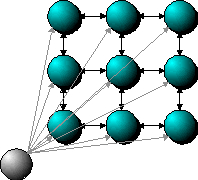Clustering & Segmentation Clustering networks add an important dimension to any data analysis effort. They are easy to use and extremely flexible.  Because of their lattice structure, they can effectively map high dimensionality vectors (i.e., many variables) into a smaller number of dimensions.  This provides an excellent structure for preprocessing and visualizing data.  They are also used for dataset sampling, outlier detection, and input validation for other types of models. Clustering models use a form of unsupervised, competitive learning.  They are “unsupervised” in that there is no “right” answer provided in order to train the model.  Instead, the model wraps itself around the dataset in order to provide coverage across the entire distribution of data.  When a new data point is provided to the model, all of the nodes compete for it. During training, the closest node wins and is adjusted closer to the data point.  After training, nodes are no longer adjusted and winning nodes are used for classification of the incoming data points.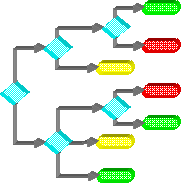Decision Trees Decision trees use hierarchical, joint variable conditions to break-up a solution space into subspaces.  These conditional sub-spaces can then be used to classify input patterns or forecast output values.  They are called trees due to the hierarchical node/link flowchart-type graph that is often used to depict the various conditional decision paths.  Tree leaf nodes can be interpreted as IF-THEN rules where each link leading into the node represents a set of AND-ed conditions (ex., IF iVar1=a and iVar2=b and iVar3=c THEN oVar = d). Decision trees train by selecting the most discriminative variable from the list of candidate variables then recursively selecting the most discriminative variable for each branch (i.e., possible value) of the previous variable.  Training stops when the are no further variables can be added to improve classification accuracy or tree branches become overly specific (i.e., cover too few cases).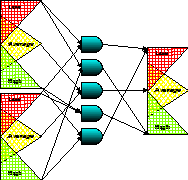Fuzzy Logic Fuzzy logic provides an intuitive, rule-based means for expressing continuous-valued or proportional relationships between variables.  Variable values are defined to fall within membership functions to a greater or lesser degree (Ex., Container is Empty, Price is High, Inventory is Low).  Fuzzy IF-THEN rules use logical operators to combine joint variable memberships to express continuous, logical rule behavior.  When a fuzzy system is executed, every rule fires...but only to the degree that each rule's premise is true.  Many rules may be true to degree 0, thus, have no effect on the outcome.  Fuzzy logic is a fit for problems where there is a good understanding of the system's dynamics such that meaningful rules can be written, even if they are imprecise.  Once the rules are defined, rule weights can be tuned with available data.  Because variable memberships can overlap with one another, fuzzy systems can be more robust than crisp rule-based approaches.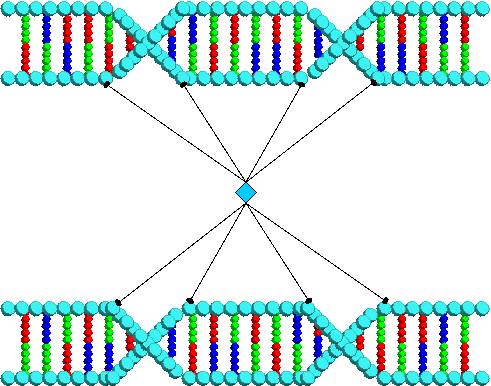Genetic Algorithms Genetic algorithm technology is a powerful optimization method.  It’s name comes from the use of genetically analogous search operators such as cross-over and mutation, and principles of survival of the fittest.  With GA, a population of solutions is generated and evaluated as to each solution’s “fitness”.  Then, each solution’s characteristics are probabilistically carried over into the next generation based on how well it solves the problem. GA technology has proven to be a very powerful generalized optimization method.  It is extremely flexible and can be used to optimize complex computational structures.